The fundamentals off: "How do Mechanical Calculators count?"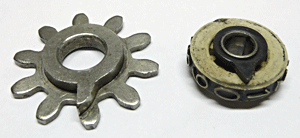Gears with a zero ridge
Subtracting (and .....  avoid nine complements notation on unbalanced numbers)
Subtraction is done by turning the gear in the opposite direction, this can be done by putting the gear rack on the tooth rack not on the extension direction as by an add, but on the the revers direction, when the tooth rack turns to the rest position. But imagine what the result will be on a gear, with 0 to nine figures, when you subtract one from zero. A nine will occur, the so called nine complement presentation.
Later machines have therefor an extra gear rack which is connected with the result register and put on the tooth rack when the result passes zero. The result register will turn the opposite direction and an one will be displayed. An sign will off coarse as well be seen, to make clear that we dealing with negative numbers.
Something like that, mechanical calculators do as well, the teeth of a gearwheel are marked with numbers, from zero to nine. But where to start on the gearwheel, so that we clearly recognize the corresponding figures of it. The start tooth, the zero one, is marked with an extra ridge on the side and start to count from there on.
Now the question occurs: "Do they count clockwise or counter clockwise"? In fact they do both. But it doesn't matter, as long as they stick to the one throughout the whole design. The gearwheels used, for displaying the results, mounted number wheels on the side and show the numbers via an window.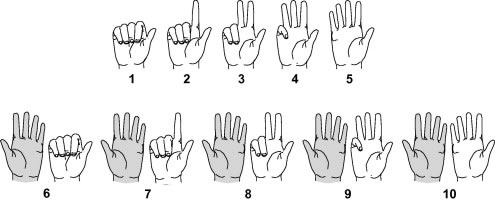Mechanical calculators have one thing in common, they use gearwheels to count. If you are surprised, please keep in mind that in the days mechanical calculators have been developed (started 1850), microelectronics didn't exist, not even the transistor was invented yet.
Counting was done on paper, they used the abacus or their fingers to count. Mostly starting to mark their thumb as the "one" and count on with the other fingers, as showed to the left.

Multiply and divide

A multiplication is performed, in fact by multiple addings, an division by multiple subtractions. The mechanisms to control these operations differ per calculation technology and will be pointed out in the next chapters, per technology. But key here is shifting of the input and or result registers. This is done by the carriage at the machine below. Keep in mind that shifting to, for instance, an higher position in a register, is in fact multiply this register content by ten. Shift it a second time is multiplying it by hundred, etc.
The procedure for a multiply:

• set the key board with the multiplicand for instance 25.
• add this for instance 5 times to the result register on the carriage
• shift the carriage on position to the right
• add the multiplicand 2 times.
• shift and add 2 times , shift and add 5 times
• the result is 5225 times the multiplicand 25. (please see video below)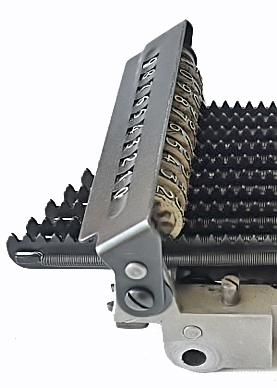Back to the gears used to show the numbers. The picture to the right shows an other example of moving an tooth rack to update the gear content. As well from a key board. You see a gear now in a rack configuration and the metal window, displaying the complete number. Each digit one gear. All the mechanical calculators use this principle, in one way or the other, however they differ in feeding these gearwheels during the calculations, some have cylindrical tooth configurations etc.
But for now it is clear that the extension of the tooth racks turns the gears. Here the gears are fixed on the tooth racks, but imagine that they can be pulled from the racks and put them back on again. This is used by for instance an add. (please see the two pictures and follow the sequence at the video below)

• the gears are put on the tooth rack (all zero's on the first picture)
• we shift the first digit tooth rack two teeth (the red arrow, on the second picture)
• we pulled gear of the first digit from the tooth rack and shift the tooth rack to the zero position again (no picture)
• put the gear on the tooth rack again for the eventual next. (no picture)
• this all results in an add of two, to the first digit.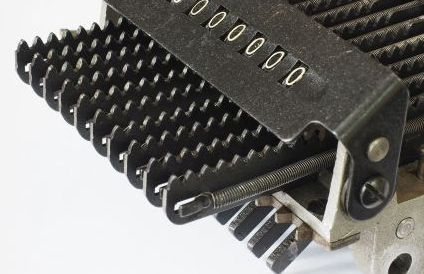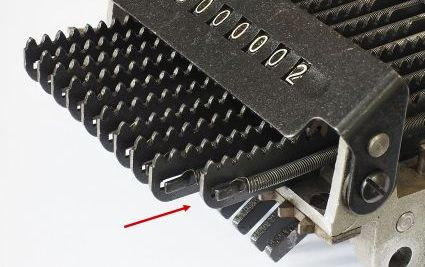This is an shifting of the carriage example, useful applied at an multiple add, or the multiply of 5225 times 25.
When a machine is capable of performing multiplications and dividing automatically, it needs extra registers to store and a counter to keep track of the number of addings and subtractions. For multiplications the counter counts down per digit and at a dividing we don't know the number of subtractions yet, so we count these subtractions with an up counter. The number window to the left is such a register. At the end of this calculation it shows the multiplier 5225.
More details at the calculating technologies.

12345 Multiplied by 121
000012345 one times the units
000123450 two times the ten numbers
000123450
001234500 one times the hundreds
001493745 the result

675 divided by 25
(multiple subtractions)
000675
-00250 = 00425 first subtraction
-        00250 = 00175 second subtr.
-                00025 = 150
and so on, goes in total 7 times. results in 27

Key board (1)
An basic example of how a gear is turned in mechanical calculators is for instance at a key board. There results a push on a key in an shifting out of a metal plate, including an tooth rack. This extension is an equivalent to the pushed figure. This transition of an extension of a tooth rack, as drive of gears, we see on many places in mechanical calculators.

Here we see this sequence, manually carried out, at the internal ASCOTA calculating unit. You see two gear rack above each other. In fact there are three, one is hidden. The result register is on top. Why three? This is to provide us with correct presentation of negative numbers. Key word here is nine complement. Please see later on at the subtraction chapter.
You might have noticed the ridges between the result register gears. These are used to generate a the tens carry, an add where the nine passed.

How a crank or a motor activate the mechanisms

We are now entering the machine control center, the main cam shaft, to show other examples off how movements are generated to control mechanisms. These shafts constitute the main sequential control / the heart of the machine. Special shaped cam plates, have cam levers / followers, which pressed and holds against the side of the cams.
You might conclude: Nearly all activities in mechanical calculators are controlled by cam plates and cam levers. For the functional design the diagram of activities as function of the shaft angle is all decisive. Please click here to get the complete picture.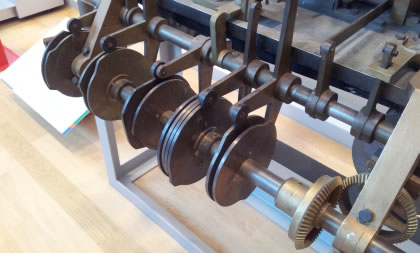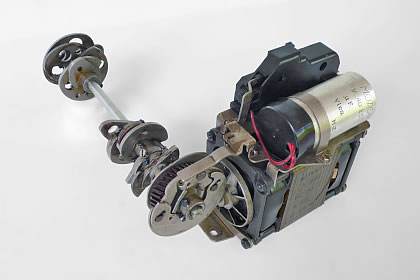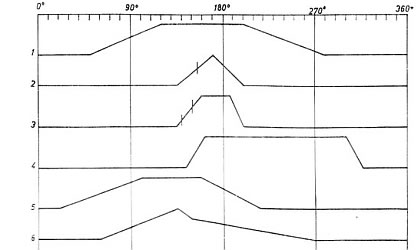Key boards (2)

So far we saw the calculation mechanisms. Now a little more on the eye catching parts of the early mechanical calculators, the key-boards. These machines have a matrix of key's.
The vertical key position represents the value (1-9) for inputting the value of each digit. The zero is default when no key is pressed.
The horizontal key position represents the digits / decades. The right most are the units, then the tens, the hundreds, the thousands etc.
The used keyboard mechanism differs per manufacturer and calculating technology but the general function is converting a pressed key to an extension of a bar or pawl equivalent to the key value. This extension has to turn a gear on a certain angle or push a pinion a certain distance. More details at the several mechanisms at the calculating technologies at the menu to the left.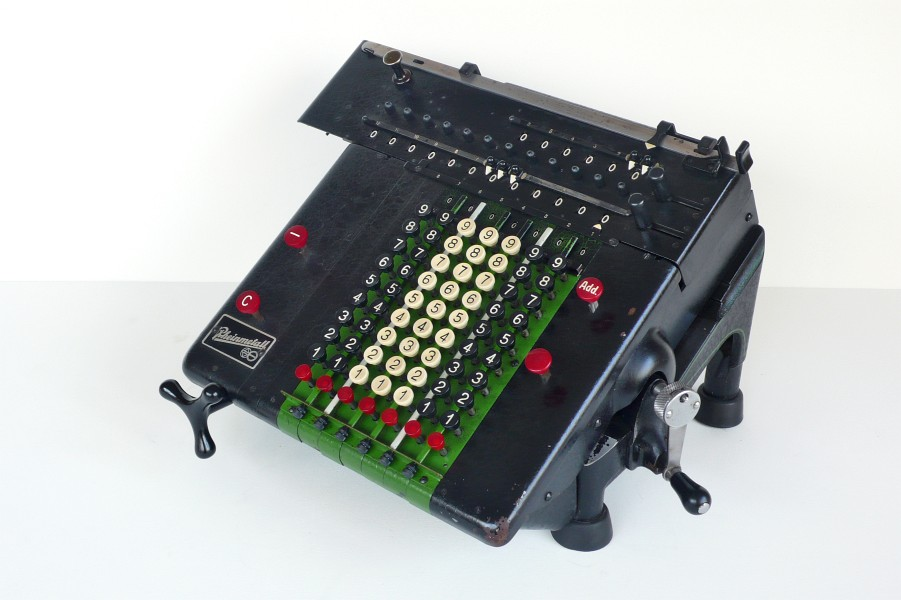The emphasis here is not add or subtract, but the inverting turning direction by the swinging of gear racks. Later on in the video you see the minus sign is controlled by the left tooth rack. The last clip shows the two plates above the register who fixed the gear content or prevent that the content is changed during the shift.

To the right schematic of the tooth rack is shown. The bottom line is the tooth rack, the red circle the result register and the green and blue circles are the extra gear racks.
You will understand that this is just an implementation of these functions from one manufacturer, others implement these differently.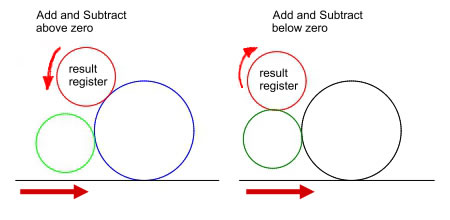Tens carry or borrow
Lets say we like to add six with seven. Now we enter first 6 and then add seven. In the meantime we exceed transition from nine to ten, an "tens carry" has to be carried out. The next digit should be increased by one. At an subtraction we have to borrow from the higher digit when the result passes the zero.
There various way's this is implemented but on the mechanisms are far in the cellar of the machines or at the carriages. From the last is on the right an example.
To understand what's happen here keep in mind that row to look at is de zeros an nine. In a previous add the nine is generated. This has set an glider (middle row of the video). When an one is added, the zero turns to zero, and an pin is following the glider to the next digit to increase that second digit. When that is happened the glides is pushed away by the bigger knock.